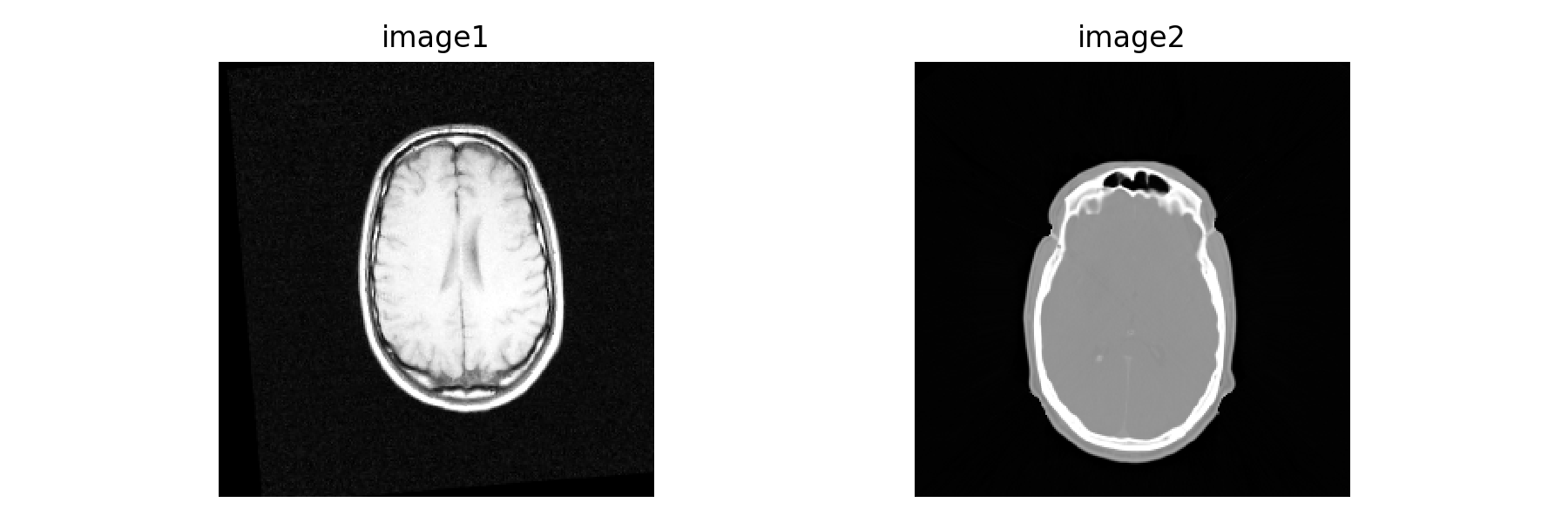# Visualization of Segmentation and Registration Results

In this notebook we illustrate various ways one can display the results of segmentation and registration algorithms so that they can be easily incorporated into a manuscript. For interactive data exploration we recommend using dedicated programs (e.g. 3D slicer).

Two key points to remember when working with bio-medical images:

1. Most often images have a high dynamic range. Thus, to write them to file in a format appropriate for use in a manuscript we will need to map the intensities to a low dynamic range (e.g. [0,255]). In SimpleITK this is readily done with the IntensityWindowingImageFilter.
2. Images may have non-isotropic spacing between pixels. The file formats appropriate for use in a manuscript (e.g. png, jpg) assume isotropic pixel spacing. This requires that we resample the image before writing to disk. The function make_isotropic in the code cell bellow resolves this issue.

The following filters and their procedural counterparts are useful for various image creation tasks, as illustrated in this notebook:

In :
%matplotlib notebook

import numpy as np

import SimpleITK as sitk
# Utility method that either downloads data from the Girder repository or

import gui

# Always write output to a separate directory, we don't want to pollute the source directory.
import os
OUTPUT_DIR = 'Output'

def make_isotropic(image, interpolator = sitk.sitkLinear):
'''
Resample an image to isotropic pixels (using smallest spacing from original) and save to file. Many file formats
(jpg, png,...) expect the pixels to be isotropic. By default the function uses a linear interpolator. For
label images one should use the sitkNearestNeighbor interpolator so as not to introduce non-existant labels.
'''
original_spacing = image.GetSpacing()
# Image is already isotropic, just return a copy.
if all(spc == original_spacing for spc in original_spacing):
return sitk.Image(image)
# Make image isotropic via resampling.
original_size = image.GetSize()
min_spacing = min(original_spacing)
new_spacing = [min_spacing]*image.GetDimension()
new_size = [int(round(osz*ospc/min_spacing)) for osz,ospc in zip(original_size, original_spacing)]
return sitk.Resample(image, new_size, sitk.Transform(), interpolator,
image.GetOrigin(), new_spacing, image.GetDirection(), 0,
image.GetPixelID())


# Combining two images¶

There are a variety of ways we can overlay two (partially) overlapping images onto each other. The common approaches include:

1. Use of alpha blending.
2. Use of a checkerboard pattern with the pixel values in adjacent squares/boxes taken from each of the images.
3. When the pixel values are scalars (gray scale images), combine the two images in different channels, resulting in a color image.

We will start by loading two images whose content luckily overlaps in physical space. Before we can combine the two, we need to resample one of them so that they both occupy the same spatial region. In addition we should also rescale the intensities so that they occupy the same range. In our case we will map them to [0,255], based on the desired windowing.

In :
img1 = sitk.ReadImage(fdata("training_001_mr_T1.mha"))
img2 = sitk.Resample(img2_original, img1)

# Obtain foreground masks for the two images using Otsu thresholding, we use these later on.
msk1 = sitk.OtsuThreshold(img1,0,1)
msk2 = sitk.OtsuThreshold(img2,0,1)

gui.MultiImageDisplay(image_list = [img1, img2],
title_list = ['image1', 'image2'],
figure_size=(9,3));

Fetching training_001_mr_T1.mha
Fetching training_001_ct.mhaIn :
# Having identified the desired intensity range for each of the
# images using the GUI above, we use these values to perform intensity windowing and map the intensity values
# to [0,255] and cast to 8-bit unsigned int
img1_255 = sitk.Cast(sitk.IntensityWindowing(img1, windowMinimum=2, windowMaximum=657,
outputMinimum=0.0, outputMaximum=255.0), sitk.sitkUInt8)
img2_255 = sitk.Cast(sitk.IntensityWindowing(img2, windowMinimum=-1018, windowMaximum=1126,
outputMinimum=0.0, outputMaximum=255.0), sitk.sitkUInt8)


## Alpha blending¶

Alpha blending combines the pixels from the two images as follows: $$I_{output} = \alpha I_1 + (1-\alpha)I_2,\;\;\; \alpha \in[0.0,1.0]$$

When our images consist of a foreground and background we can use alpha blending in a manner that takes this into account. Instead of blending all of the pixels using the formula above, we use this formula only in the regions where the foregrounds overlap. In regions where the foreground from one image overlaps with the background of the other we simply copy the foreground. This improves visibility as we are not blending a region that contains information with an empty region.

The code below allows us to experiment with various alpha blending strategies.

In :
def mask_image_multiply(mask, image):
components_per_pixel = image.GetNumberOfComponentsPerPixel()
if  components_per_pixel == 1:
else:
return sitk.Compose([mask*sitk.VectorIndexSelectionCast(image,channel) for channel in range(components_per_pixel)])

'''
Alaph blend two images, pixels can be scalars or vectors.
The region that is alpha blended is controled by the given masks.
'''

mask1 = sitk.Image(image1.GetSize(), sitk.sitkFloat32) + 1.0
else:
else:

components_per_pixel = image1.GetNumberOfComponentsPerPixel()
if components_per_pixel>1:
img1 = sitk.Cast(image1, sitk.sitkVectorFloat32)
img2 = sitk.Cast(image2, sitk.sitkVectorFloat32)
else:
img1 = sitk.Cast(image1, sitk.sitkFloat32)
img2 = sitk.Cast(image2, sitk.sitkFloat32)



We now create 3D images using all four combinations of alpha-blending and masks. As we are working with a 3D image and we want to save it as a figure for use in a manuscript, we will create a 2D montage image using the axial slices from the volumes.

In :
# Combine the two volumes
images_list = [(alpha_blend(img1_255, img2_255), 'alpha_blend_standard'),

# Tile the volumes using the x-y plane (axial slices)
all_montages = []
for img,img_name in images_list:
num_slices = img.GetDepth()
tile_w = int(np.sqrt(num_slices))
tile_h = int(np.ceil(num_slices/tile_w))
tile_image = sitk.Tile([img[:,:,i] for i in range(num_slices)], (tile_w, tile_h))
sitk.WriteImage(sitk.Cast(tile_image, sitk.sitkUInt8), os.path.join(OUTPUT_DIR,img_name+'.png'))
all_montages.append(tile_image)

# Display all montages by combining them into a faux volume. Notice that scrolling through this
# volume creates the illusion of motion due to the change in intensities (the interested
# reader is referred to "Visual dissociations of movement, position, and stereo depth: Some phenomenal
# phenomena", R. L. Gregory, P. F. Heard).
gui.MultiImageDisplay(image_list = [sitk.JoinSeries(all_montages)],
title_list = ['Montages With Different Alpha Blending Strategies'],
figure_size=(8,8));